# Multiply Formula In ExcelArticle byExcelMojo Team## What Is Multiply Formula In Excel?

The Multiply Formula in Excel helps users find the product of numeric values. It is the basic mathematical multiplication of multiplying 2 or more numbers in Excel using the “=” and the “*” signs.

The Multiply Excel Formula has an alternative inbuilt “Math & Trig” function, “PRODUCT”, so we can insert the formula from the “Function Library” or enter it directly in the worksheet.

For example, the image below depicts the Value in columns A and B. We will use the basic multiplication method to calculate the product.

Select cell C2, enter the formula =A2*B2, and press the “Enter” key.

The result is ‘4’, as shown above.

###### Key Takeaways
• The Multiply formula in Excel helps use multiply multiple numeric values.
• Although not a predefined function, the alternative PRODUCT function or the basic asterisk (*) method performs the required calculations.
• If any of the selected cell values are empty or blank, by default, the formula considers it as 0, and the final output will certainly be 0. However, in such scenarios, if one of the selected cell values is alpha-numeric, we will get an error.
• Both the methods, the “*” asterisk formula and the PRODUCT function, accept only numeric input.

### Multiply() Excel Formula

There are two ways to multiply, i.e., using the basic or the direct method or the Product().

1. The basic method’s mathematical expression is =number1 * number2 * number3…
• number: It is a mandatory argument that is the numeric value.
2. The syntax of the Multiply() Excel formula of PRODUCT() is,

The argument of the Multiply() Excel formula of PRODUCT() is,

• number: It is a mandatory argument. The number that we want to multiply.

### How To Use Multiply Excel Formulas?

We can use the Multiple Excel Formula of the PRODUCT() function in 2 ways, namely:

1. Access from the Excel ribbon.
2. Enter in the worksheet manually.

#### Method #1 – Access from the Excel ribbon

Choose an empty cell → select the “Formulas” tab → go to the “Function Library” group → click the “Math & Trig” option drop-down → select the “PRODUCT” function, as shown below.

The “Function Arguments” window appears. Enter the arguments in the “Number1” & “Number2”, and click “OK”, as shown below.

#### Method #2 – Enter in the worksheet manually

1. Select an empty cell for the output.
2. Type =PRODUCT( in the selected cell. [Alternatively, type =P or =PR and double-click and then the PRODUCT excel function from the list of suggestions shown by Excel.]
3. Enter the arguments as cell values or cell references in excel.
4. Press the “Enter” key.

The succeeding example depicts the values, and we will multiply the given values using the basic “*” asterisk method.

In the table, the data is,

• Column A contains Value 1.
• Column B contains Value 2.
• Column C contains the Output.

The steps to calculate using the PRODUCT formula to Multiply in excel are as follows:

1. Select cell C2, and enter the formula =A2, i.e., the first value.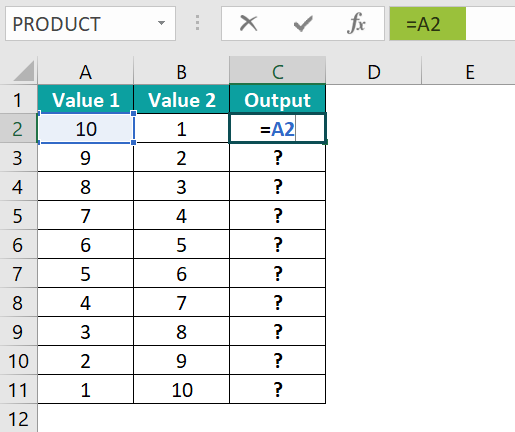2. Enter “*B2”, i.e., the second value. The entered complete formula is = A2 * B2.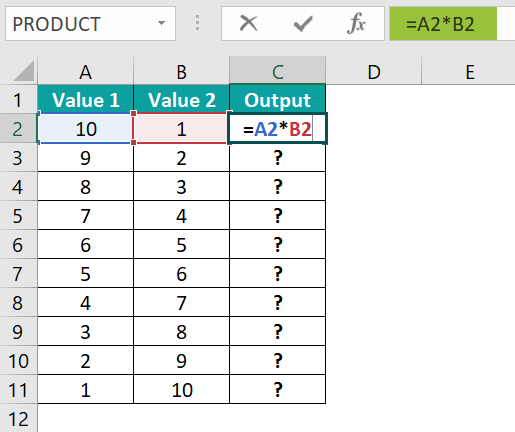3. Press the “Enter” key. The result is “10”, as shown below.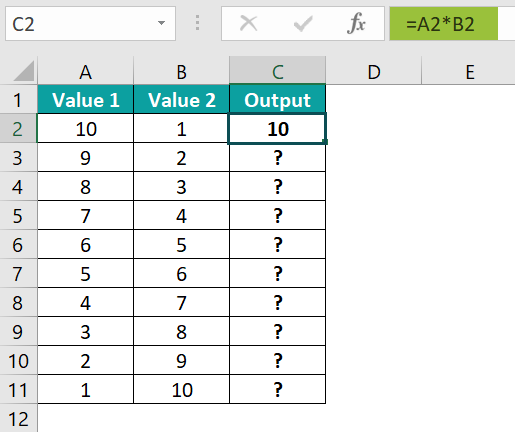4. Drag the formula from cell C2 to C11 using the fill handle. The output is shown below.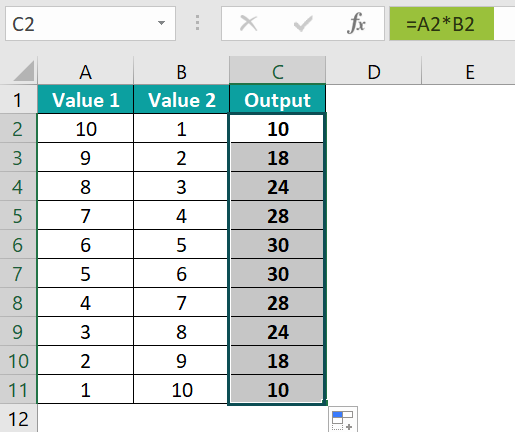### Examples

We will consider advanced scenarios using the PRODUCT Multiply Formula in Excel examples.

#### Example #1

The succeeding example depicts the values, and we will multiply the given values using the PRODUCT Excel Function.

In the table, the data is,

• Column A contains Value 1.
• Column B contains Value 2.
• Column C contains the Output.

The steps to calculate using the PRODUCT formula are as follows:

• Step 1: Select cell C2, and enter the formula =PRODUCT(A2¸ i.e., the first value.
• Step 2: Enter the value of ‘number2’ as B2, and close the brackets. The complete formula is =PRODUCT(A2,B2).
• Step 3: Press the “Enter” key. The result is “1”, as shown below.
• Step 4: Drag the formula from cell C2 to C11 using the fill handle. The output is shown below.

#### Example #2

The succeeding example depicts the values, and we will calculate the percentage of the given values using the “*” basic asterisk Formula by multiplying them with the values.

In the table, the data is,

• Column A contains Value 1.
• Column B contains the Output.

The steps to calculate the value by the “*” basic asterisk Formula are as follows:

• Step 1: Select cell B2, enter the formula = A2 * 12%, and press the “Enter” key. The result is cell “5.4”, as shown below.
• Step 2: Drag the formula from cell B2 to B6 using the excel fill handle. The output is shown below.

#### Example #3

The succeeding example depicts the values, and we will multiply the given values using the “*” basic asterisk Formula.

In the table, the data is,

• Row 1 contains Value 1.
• Row 2 contains Value 2.
• Row 3 contains the Output.

The steps to calculate the value by the “*” basic asterisk Formula are as follows:

• Step 1: Select cell B3, enter the formula =B1*B2, and press the “Enter” key. The result is “478742550”, as shown below.
• Step 2: Drag the formula from cell B2 to E2 using the fill handle. The output is shown below.

### Important Things To Note

• The “#VALUE!” error occurs when,
• The non-numeric values are entered.
• One value is non-numeric, and the other value is blank, empty, or 0.
• When using the Basic asterisk formula method, always ensure to start with the “=” sign, else we will not get the calculated result, but the entry will remain as it is.

1. What is the Multiply formula in Excel?

There is no specific multiplication formula in Excel. Instead, we use the asterisk “*” and the PRODUCT function to perform multiplication.

The formula using the “*” asterisk method is =number 1 * number 2

The syntax for the PRODUCT function is =PRODUCT(number1,[number2], …)

2. How to multiply more than two columns in Excel using the “*” asterisk?

For example, the image depicts the values, and we will calculate the multiplication of the given values using the “*” basic asterisk Formula.

In the table, the data is,
Column A contains Value 1.
Column B contains Value 2.
Column C contains Value 3.
Column D contains the Output.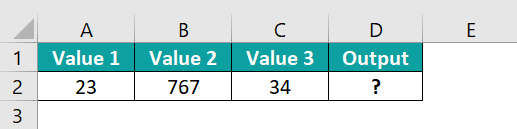The procedure to calculate the value using the “*” basic asterisk Formula is,

Select cell D2, enter the formula = A2 * B2 * C2, and press the “Enter” key.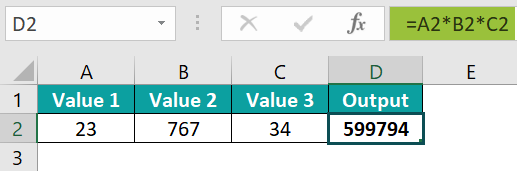The result is “599794”, as shown above.

3. How to multiply more than two columns in Excel using the PRODUCT function?

For example, the image depicts the values, and we will multiply the given values using the PRODUCT Excel Function.

In the table, the data is,
Column A contains the Value1.
Column B contains the Value2.
Column C contains Value 3.
Column D contains the Output.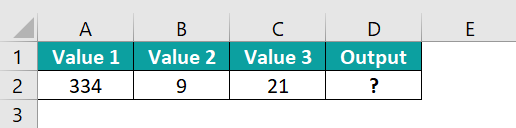The procedure to calculate the value using the PRODUCT Formula is,

Select cell D2, enter the formula =PRODUCT(A2,B2,C2), and press the “Enter” key.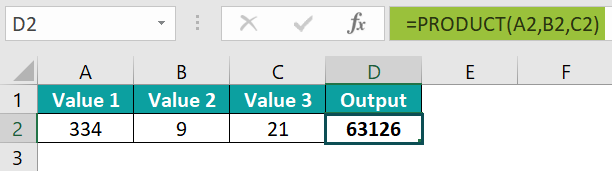The result is “63126”, as shown above.